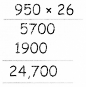Students can download 5th Maths Term 1 Chapter 2 Numbers Ex 2.7 Questions and Answers, Notes, Samacheer Kalvi 5th Maths Guide Pdf helps you to revise the complete Tamilnadu State Board New Syllabus, helps students complete homework assignments and to score high marks in board exams.

## Tamilnadu Samacheer Kalvi 5th Maths Solutions Term 1 Chapter 2 Numbers Ex 2.7

Question 1.
Multiply
a. 473 × 48
b. 4052 × 19
c. 876 × 25
d. 854 × 21
e. 417 × 39
f. 870 × 28
a. 473 × 48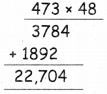b. 4052 × 19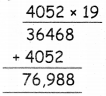c. 876 × 25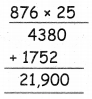d. 854 × 21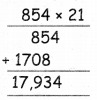e. 417 × 39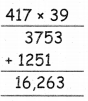f. 870 × 28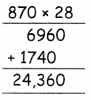Question 2.
a. There are 55 mangoes in a basket, Cost of one mango is 7 15. What is the total cost of 55 mangoes?
Total number of mangoes = 55
Cost of one mango = 7 15
∴ Total cost of 55 mangoes = 55 × 15
= ₹ 825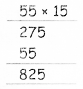b. There are 55 passengers in a bus. Each of them get tickets of 7 25. What is the amount collected by the conductor?
Total number of Passengers = 55
Amount collected for each one = ₹ 25
Total amount collected by the conductor
= 55 × 25
= ₹ 1,375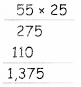c. A classroom has 23 benches, cost of one bench is 7 725. What is the total cost of all the 23 benches?
Total number of benches = 23
Cost of one bench = ₹ 725
∴ Cost of 23 benches = 725 × 23
= ₹ 16,675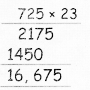d. There are 675 people living in a village. One person uses 25 l of water daily. How much of water is needed for the village for one day?
Total number of people in a village = 675
Water used by them daily = 25 litres
∴ Need of water for the village per day
= 675 × 25
= 16,875 litres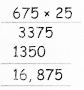e. In a building, there are 26 rooms, cost of painting for one room is ₹950. What is the total cost of painting the building?